Like   Tweet   Pin   +1   in
 /* styles */ Tai Chi Stories, Tips and Resources   ||   Issue #20 October 2019
 table div table+table+table div table{width:100%;padding:0}table div table+table+table div table img{width:96.23%;padding:0;float:none}table div table+table+table div table td{width:100%;padding:0 1.88% 18px}/* styles */table div table+table+table+table div table,table.module-3{width:3.77%;float:right;padding:0}table div table+table+table+table div table a{border:0 none;text-decoration:none}table div table+table+table+table div table img{width:100%!important;border:0 none;text-decoration:none}table div table+table+table+table div table td{width:100%;padding:0 0 20px 20px}/* styles */ Dear Reader, In this month's issue you can read Frank's story - reinforcing a belief of mine when it comes to engaging aged care residents. There are two definitions for you - Chi and Chi Kung (Qigong) - and two short, helpful videos. I share a tip I find helpful about focusing, and reflect on the importance of regular practice for any skill. I also explain a one-off presentation I'll be offering for aged care residents in 2020. Regards Chris Bennett Chris Chi
 table div table+table+table+table+table div table{width:100%;padding:0}table div table+table+table+table+table div table img{width:96.23%;padding:0;float:none}table div table+table+table+table+table div table td{width:100%;padding:0 1.88% 18px}/* styles */## Frank's Story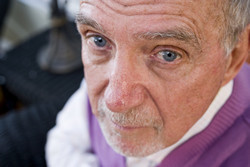Frank sat there staring at me, without moving, during our one hour Chair Chi session. He didn't participate. Just stared at me.

And that happens occasionally when I work with a group of residents. If it does, I simply continue on, hoping the person will join in eventually.

Well ... after I finished this session and was about to leave, a lifestyle staff member came up to me and said, 'That was terrific, Frank never stays for activities' ... Read More

 table div table+table+table+table+table+table+table+table div table{width:100%;padding:0}table div table+table+table+table+table+table+table+table div table img{width:96.23%;padding:0;float:none}table div table+table+table+table+table+table+table+table div table td{width:100%;padding:0 1.88% 18px}/* styles */## Chi Kung or Qigong?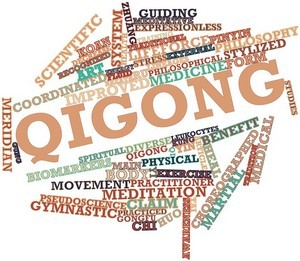The words Chi Kung and Qigong, both pronounced the same way, describe the art of cultivating one's Chi through correct body posture, breathing, and movement.

Qigong means Qi (Chi) Gong (Kung).

 table div table+table+table+table+table+table+table+table+table+table+table div table{width:100%;padding:0}table div table+table+table+table+table+table+table+table+table+table+table div table img{width:96.23%;padding:0;float:none}table div table+table+table+table+table+table+table+table+table+table+table div table td{width:100%;padding:0 1.88% 18px}/* styles */## Mindfulness: A Scientific Perspective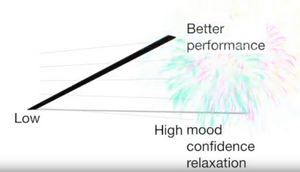Here's a short clip on 'mindfulness for sports performance A systematic review' by Mike Noetel, Australian Sport Psychologist.

He discusses meta analysis and studies regarding correlation and causation re mindfulness on performance in sport.

The studies indicate that "more mindful athletes tend to be more mentally tough, more motivated and more confident".

 table div table+table+table+table+table+table+table+table+table+table+table+table+table+table div table{width:100%;padding:0}table div table+table+table+table+table+table+table+table+table+table+table+table+table+table div table img{width:96.23%;padding:0;float:none}table div table+table+table+table+table+table+table+table+table+table+table+table+table+table div table td{width:100%;padding:0 1.88% 18px}/* styles */## Tai Chi Tip: Focus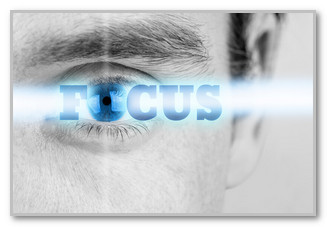Practicing Tai Chi in a quiet place is preferable but is not always possible. Sometimes noise of some sort can interfere with the meditative effects of Tai Chi.

When that happens to me, I try to reduce the effect the noise has on me by concentrating on my breathing as I practice the form.

 table div table+table+table+table+table+table+table+table+table+table+table+table+table+table+table+table+table div table{width:100%;padding:0}table div table+table+table+table+table+table+table+table+table+table+table+table+table+table+table+table+table div table img{width:96.23%;padding:0;float:none}table div table+table+table+table+table+table+table+table+table+table+table+table+table+table+table+table+table div table td{width:100%;padding:0 1.88% 18px}/* styles */## Practice What You Preach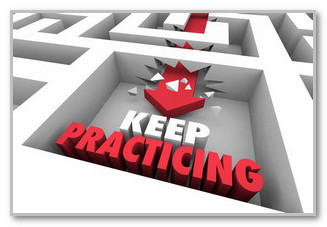I always encourage participants in my aged care and sports chi workshops to train at least three times a week so they can maintain a certain skill level they have learned.

It's something I preach and practice in my own Tai Chi training. And there is no better example than stance training to emphasise the importance of practicing three times a week to maintain a skill level.

 table div table+table+table+table+table+table+table+table+table+table+table+table+table+table+table+table+table+table+table+table div table{width:100%;padding:0}table div table+table+table+table+table+table+table+table+table+table+table+table+table+table+table+table+table+table+table+table div table img{width:96.23%;padding:0;float:none}table div table+table+table+table+table+table+table+table+table+table+table+table+table+table+table+table+table+table+table+table div table td{width:100%;padding:0 1.88% 18px}/* styles */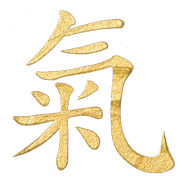CHI: The 'intrinsic energy' which circulates in all living things (Dr. Yang Jwing-Ming)
 table div table+table+table+table+table+table+table+table+table+table+table+table+table+table+table+table+table+table+table+table+table+table div table{width:100%;padding:0}table div table+table+table+table+table+table+table+table+table+table+table+table+table+table+table+table+table+table+table+table+table+table div table img{width:96.23%;padding:0;float:none}table div table+table+table+table+table+table+table+table+table+table+table+table+table+table+table+table+table+table+table+table+table+table div table td{width:100%;padding:0 1.88% 18px}/* styles */## What's New

 /* styles */ Next month I'll be contacting Melbourne aged care lifestyle co-ordinators to see if there is interest in a 30-60 minute Tai Chi presentation for their residents for 2020. I've run this special Tai Chi presentation previously and demonstrate various aspects of Tai Chi, with stories and humour to energise residents. This Tai Chi presentation is ideal for a Chinese New Year theme - or for any occasion during the year - to energise residents and leave smiles on their faces.
 table div table+table+table+table+table+table+table+table+table+table+table+table+table+table+table+table+table+table+table+table+table+table+table+table+table div table{width:100%;padding:0}table div table+table+table+table+table+table+table+table+table+table+table+table+table+table+table+table+table+table+table+table+table+table+table+table+table div table img{width:96.23%;padding:0;float:none}table div table+table+table+table+table+table+table+table+table+table+table+table+table+table+table+table+table+table+table+table+table+table+table+table+table div table td{width:100%;padding:0 1.88% 18px}/* styles */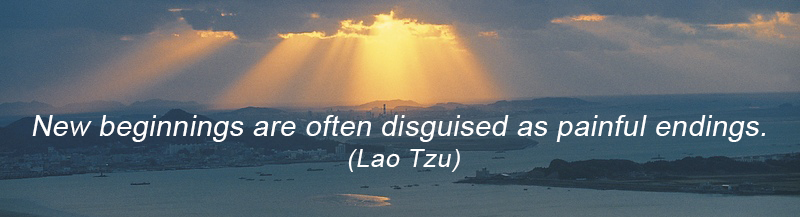table div table+table+table+table+table+table+table+table+table+table+table+table+table+table+table+table+table+table+table+table+table+table+table+table+table+table+table div table{width:100%;padding:0}table div table+table+table+table+table+table+table+table+table+table+table+table+table+table+table+table+table+table+table+table+table+table+table+table+table+table+table div table img{width:96.23%;padding:0;float:none}table div table+table+table+table+table+table+table+table+table+table+table+table+table+table+table+table+table+table+table+table+table+table+table+table+table+table+table div table td{width:100%;padding:0 1.88% 18px}/* styles *//* styles */